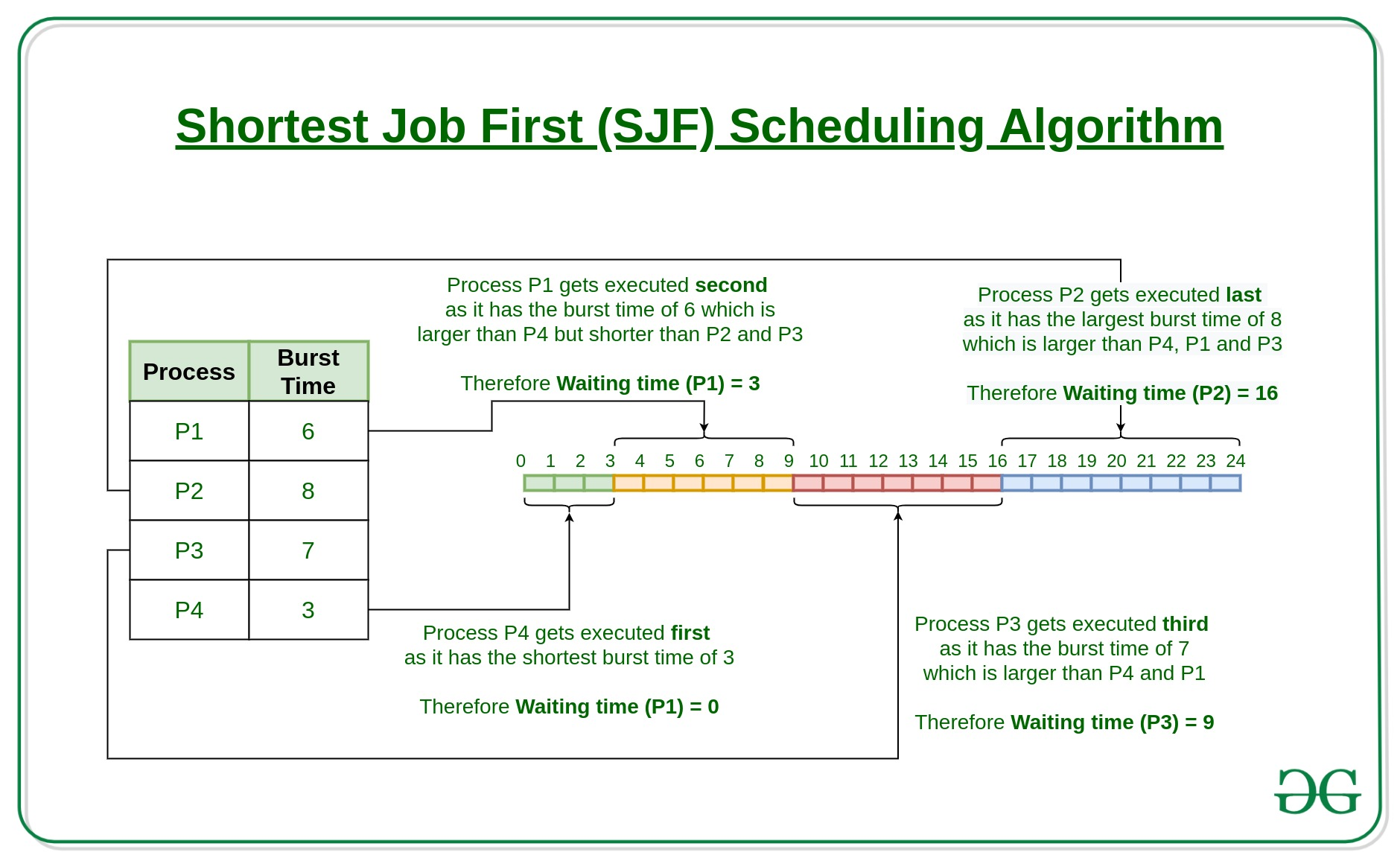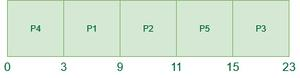# Program for Shortest Job First (or SJF) CPU Scheduling | Set 1 (Non- preemptive)

• Difficulty Level : Easy
• Last Updated : 26 May, 2022

The shortest job first (SJF) or shortest job next, is a scheduling policy that selects the waiting process with the smallest execution time to execute next. SJN, also known as Shortest Job Next (SJN), can be preemptive or non-preemptive.

Characteristics of SJF Scheduling:

• Shortest Job first has the advantage of having a minimum average waiting time among all scheduling algorithms.
• It is a Greedy Algorithm.
• It may cause starvation if shorter processes keep coming. This problem can be solved using the concept of ageing.
• It is practically infeasible as Operating System may not know burst times and therefore may not sort them. While it is not possible to predict execution time, several methods can be used to estimate the execution time for a job, such as a weighted average of previous execution times.
• SJF can be used in specialized environments where accurate estimates of running time are available.

Algorithm:

• Sort all the processes according to the arrival time.
• Then select that process that has minimum arrival time and minimum Burst time.
• After completion of the process make a pool of processes that arrives afterward till the completion of the previous process and select that process among the pool which is having minimum Burst time.How to compute below times in SJF using a program?

• Completion Time: Time at which process completes its execution.
• Turn Around Time: Time Difference between completion time and arrival time.
Turn Around Time = Completion Time – Arrival Time
• Waiting Time(W.T): Time Difference between turn around time and burst time.
Waiting Time = Turn Around Time – Burst Time

### Program for Non-Preemptive Shortest Job First  CPU Scheduling

Non-Preemptive Shortest Job First algorithm can be implemented using Segment Trees data structure. For detailed implementation of Non-Preemptive Shortest Job First scheduling algorithm, please refer: Program for Non-Preemptive Shortest Job First CPU Scheduling.

In this post, we have assumed arrival times as 0, so turn around and completion times are same.

### Examples to show working of Non-Preemptive Shortest Job First CPU Scheduling Algorithm:

Example-1: Consider the following table of arrival time and burst time for five processes P1, P2, P3, P4 and P5

The Shortest Job First CPU Scheduling Algorithm will work on the basis of steps as mentioned below:

At time = 0,

• Process P4 arrives and starts executing

At time= 1,

• Process P3 arrives.
• But, as P4 still needs 2 execution units to complete.
• Thus, P3 will wait till P4 gets executed.

At time =2,

• Process P1 arrives and is added to the waiting table
• P4 will continue its execution.

At time = 3,

• Process P4 will finish its execution.
• Then, the burst time of P3 and P1 is compared.
• Process P1 is executed because its burst time is less as compared to P3.

At time = 4,

• Process P5 arrives and is added to the waiting Table.
• P1 will continue execution.

At time = 5,

• Process P2 arrives and is added to the waiting Table.
• P1 will continue execution.

At time = 6,

• Process P1 will finish its execution.
• The burst time of P3, P5, and P2 is compared.
• Process P2 is executed because its burst time is the lowest among all.

At time=9,

• Process P2 is executing and P3 and P5 are in the waiting Table.

At time = 11,

• The execution of Process P2 will be done.
• The burst time of P3 and P5 is compared.
• Process P5 is executed because its burst time is lower than P3.

At time = 15,

• Process P5 will finish its execution.

At time = 23,

• Process P3 will finish its execution.
• The overall execution of the processes will be as shown below:

Gantt chart for above execution:Gantt chart

Now, let’s calculate the average waiting time for above example:

P4 = 0 – 0 = 0

P1 = 3 – 2 = 1

P2 = 9 – 5 = 4

P5 = 11 – 4 = 7

P3 = 15 – 1 = 14

Average Waiting Time = 0 + 1 + 4 + 7 + 14/5 = 26/5 = 5.2

• SJF is better than the First come first serve(FCFS) algorithm as it reduces the average waiting time.
• SJF is generally used for long term scheduling
• It is suitable for the jobs running in batches, where run times are already known.
• SJF is probably optimal in terms of average turnaround time.

• SJF may cause very long turn-around times or starvation.
• In SJF job completion time must be known earlier, but sometimes it is hard to predict.
• Sometimes, it is complicated to predict the length of the upcoming CPU request.
• It leads to the starvation that does not reduce average turnaround time.

## C

 `#include ` `int` `main()` `{` `    ``int` `A; ``// Matrix for storing Process Id, Burst` `                   ``// Time, Average Waiting Time & Average` `                   ``// Turn Around Time.` `    ``int` `i, j, n, total = 0, index, temp;` `    ``float` `avg_wt, avg_tat;` `    ``printf``(``"Enter number of process: "``);` `    ``scanf``(``"%d"``, &n);` `    ``printf``(``"Enter Burst Time:\n"``);` `    ``// User Input Burst Time and alloting Process Id.` `    ``for` `(i = 0; i < n; i++) {` `        ``printf``(``"P%d: "``, i + 1);` `        ``scanf``(``"%d"``, &A[i]);` `        ``A[i] = i + 1;` `    ``}` `    ``// Sorting process according to their Burst Time.` `    ``for` `(i = 0; i < n; i++) {` `        ``index = i;` `        ``for` `(j = i + 1; j < n; j++)` `            ``if` `(A[j] < A[index])` `                ``index = j;` `        ``temp = A[i];` `        ``A[i] = A[index];` `        ``A[index] = temp;`   `        ``temp = A[i];` `        ``A[i] = A[index];` `        ``A[index] = temp;` `    ``}` `    ``A = 0;` `    ``// Calculation of Waiting Times` `    ``for` `(i = 1; i < n; i++) {` `        ``A[i] = 0;` `        ``for` `(j = 0; j < i; j++)` `            ``A[i] += A[j];` `        ``total += A[i];` `    ``}` `    ``avg_wt = (``float``)total / n;` `    ``total = 0;` `    ``printf``(``"P     BT     WT     TAT\n"``);` `    ``// Calculation of Turn Around Time and printing the` `    ``// data.` `    ``for` `(i = 0; i < n; i++) {` `        ``A[i] = A[i] + A[i];` `        ``total += A[i];` `        ``printf``(``"P%d     %d     %d      %d\n"``, A[i],` `               ``A[i], A[i], A[i]);` `    ``}` `    ``avg_tat = (``float``)total / n;` `    ``printf``(``"Average Waiting Time= %f"``, avg_wt);` `    ``printf``(``"\nAverage Turnaround Time= %f"``, avg_tat);` `}`

Note: In this post, we have assumed arrival times as 0, so turn around and completion times are same.

In Set-2 we will discuss the preemptive version of SJF i.e. Shortest Remaining Time First

This article is contributed by Mahesh Kumar(NCE, Chandi). If you like GeeksforGeeks and would like to contribute, you can also write an article using write.geeksforgeeks.org or mail your article to review-team@geeksforgeeks.org. See your article appearing on the GeeksforGeeks main page and help other Geeks.